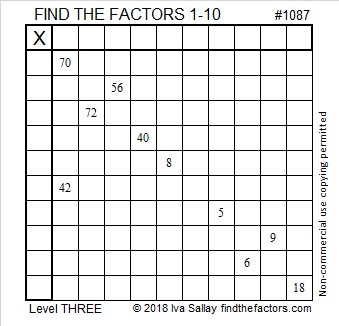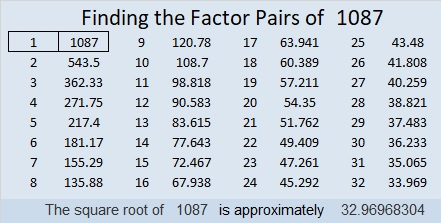# 1087 and Level 3

Using logic, start with the clue on the top row and work yourself down row by row filling in the appropriate factors while you go. You might find this level 3 puzzle a little tricky near the bottom of the puzzle, so I didn’t want to wait to share it with you. Happy factoring!Print the puzzles or type the solution in this excel file: 10-factors-1087-1094

1087 is the first prime since 1069, which was 18 numbers ago! What else can I tell you about it?

• 1087 is a prime number.
• Prime factorization: 1087 is prime.
• The exponent of prime number 1087 is 1. Adding 1 to that exponent we get (1 + 1) = 2. Therefore 1087 has exactly 2 factors.
• Factors of 1087: 1, 1087
• Factor pairs: 1087 = 1 × 1087
• 1087 has no square factors that allow its square root to be simplified. √1087 ≈ 32.96968

How do we know that 1087 is a prime number? If 1087 were not a prime number, then it would be divisible by at least one prime number less than or equal to √1087 ≈ 33. Since 1087 cannot be divided evenly by 2, 3, 5, 7, 11, 13, 17, 19, 23, 29 or 31, we know that 1087 is a prime number.1087 is also palindrome 767 in BASE 12 because
7(12²) + 6(12) + 7(1) = 1087